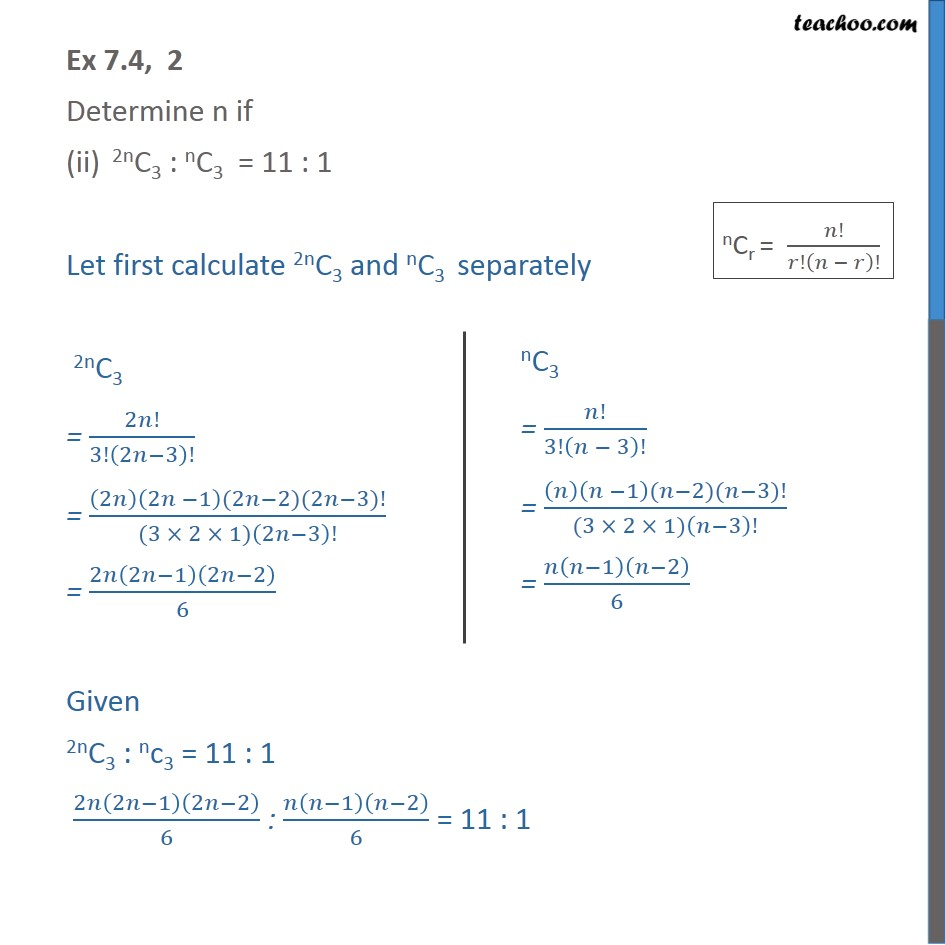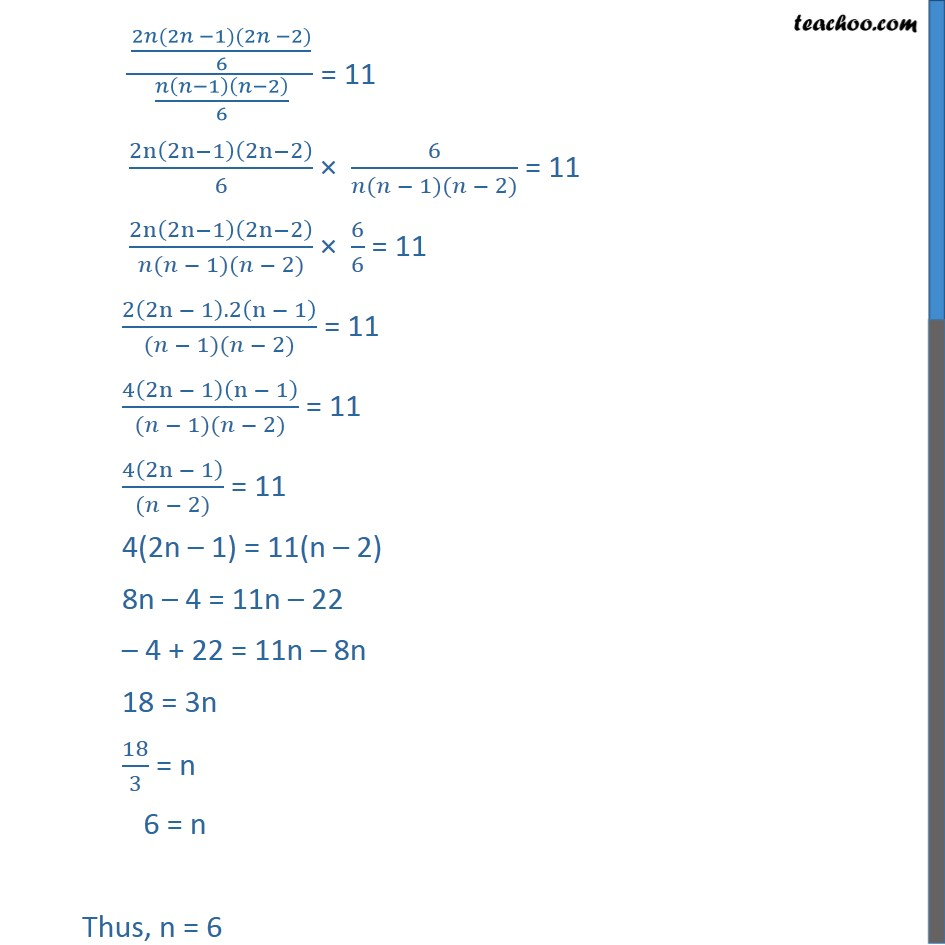Ex 6.4

Chapter 6 Class 11 Permutations and Combinations
Serial order wiseLearn in your speed, with individual attention - Teachoo Maths 1-on-1 Class

### Transcript

Ex 6.4, 2 Determine n if (ii) 2nC3 : nC3 = 11 : 1 Let first calculate 2nC3 and nC3 separately 2nC3 = 2 !/3!(2 3)! = ((2 )(2 1)(2 2)(2 3)!)/((3 2 1)(2 3)!) = 2 (2 1)(2 2)/6 Given 2nC3 : nc3 = 11 : 1 2 (2 1)(2 2)/6 : ( 1)( 2)/6 = 11 : 1 2 (2 1)(2 2)/6 : ( 1)( 2)/6 = 11 : 1 ((2 (2 1)(2 2))/6)/( ( 1)( 2)/6) = 11 2n(2n 1)(2n 2)/6 6/( ( 1)( 2)) = 11 2n(2n 1)(2n 2)/( ( 1)( 2)) 6/6 = 11 (2(2n 1). 2(n 1))/(( 1)( 2)) = 11 4(2n 1)(n 1)/(( 1)( 2)) = 11 4(2n 1)/(( 2)) = 11 4(2n 1) = 11(n 2) 8n 4 = 11n 22 4 + 22 = 11n 8n 18 = 3n 18/3 = n 6 = n Thus, n = 6